# 【扑克反水】你知道哪些牌可以4bet吗？德州扑克顶级牌手这样做

01

02

1. 将诈唬牌加入到我们的4bet范围。

2. 4bet到一个使对手处境艰难的额度。

03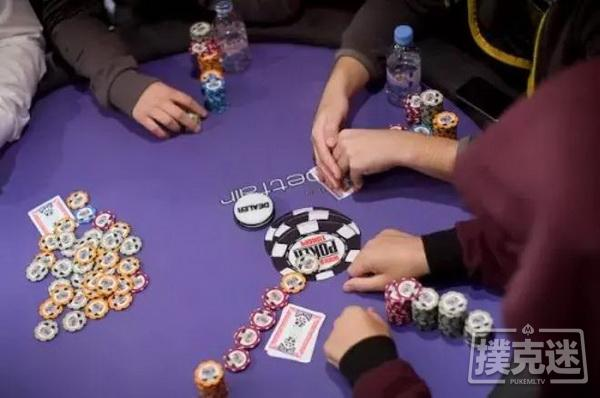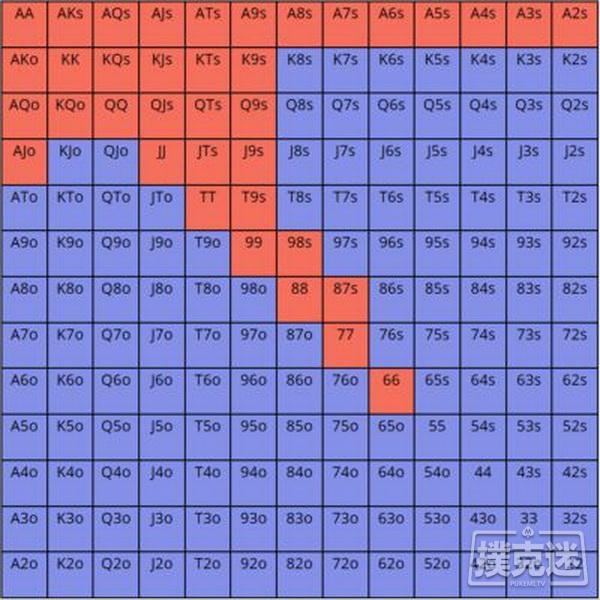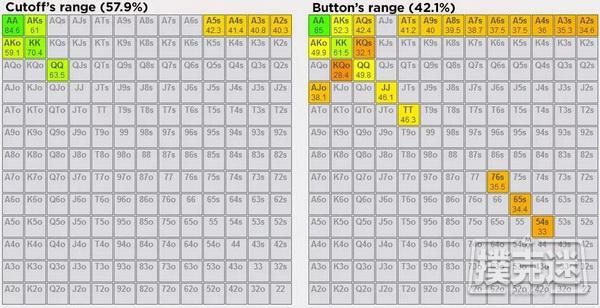X表示我们求解的下注尺度

(X – 9) / (2X + 1) = 0.3

X – 9 = 0.6X + 0.3

0.4X = 9.3

X = 23.25BB或3bet尺度的2.6倍

F表示小盲玩家必须以范围中多少百分比的部分防守。

F = 1 – (23.25 – 2.5) / (23.25 + 9 + 1)

F = 1 – 20.75 / 33.25

F = 1 – 0.62

F = 0.38 即他3bet范围中的38%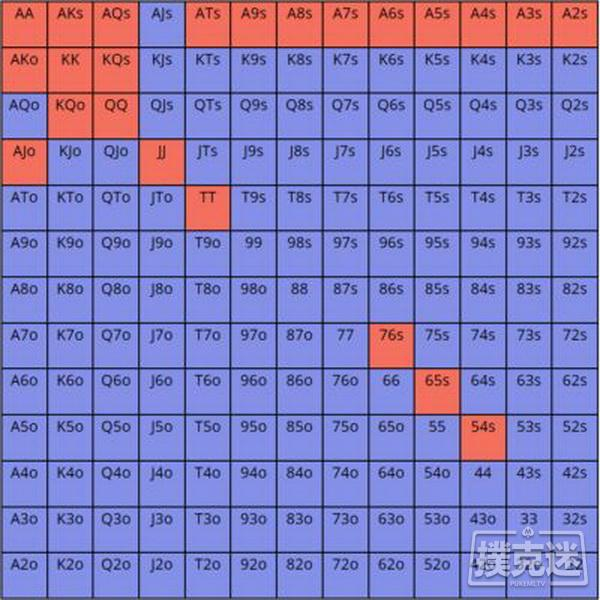CO玩家的4bet范围可能看起来是这样：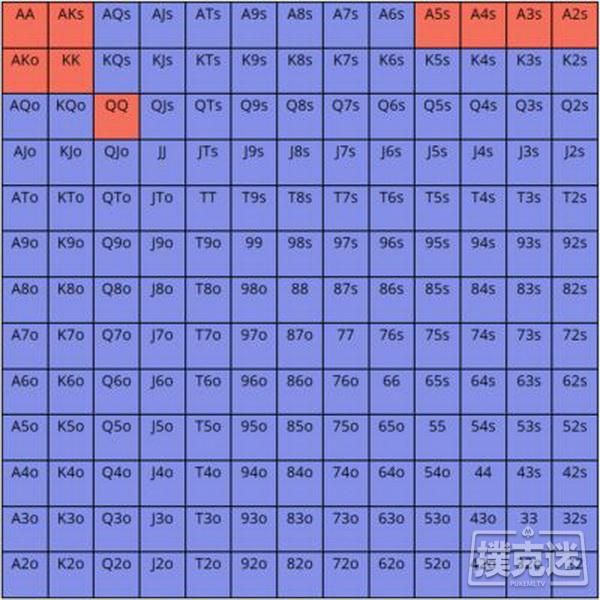X表示我们求解的下注尺度。

0.32 = (X – 9) / (2X + 1.5)

0.64X + 0.48 = X – 9

9.48 = 0.36X

X = 26.3BB或3bet尺度的2.92倍

F代表按钮玩家需要用范围中多少百分比的部分防守。

F = 1 – (26.3 – 2.5) / (26.3 + 9 + 1.5)

F = 1 – 0.65

F = 0.35 即他3bet范围中的35%

http://www.bogoupoker.com